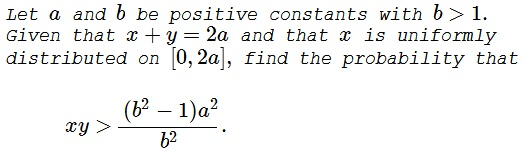# Probability of a Random Inequality

### Problem### Solution 1

Substituting $y=2a-x$ into the inequality gives

\displaystyle \begin{align} &b^2x(2a-x)\gt (b^2-1)a^2,&\text{ or,}\\ &b^2x^2-2ab^2x+(b^2-1)a^2\lt 0,&\text{ or,}\\ &(bx-(b-1)a)(bx-(b+1)a)\lt 0,&\text{ or,}\\ &\frac{(b-1)a}{b}\lt x\lt\frac{(b+1)a}{b}. \end{align}

The length of the interval where the given inequality holds is $\displaystyle \frac{(b+1)a}{b}-\frac{(b-1)a}{b}=\frac{2a}{b}.$ The sought probability is the ratio of that length to the length of the interval of the distribution of $x,$ i.e. $2a.$ Thus, we have $\displaystyle \frac{2a}{b}\div 2a=\frac{1}{b}.$

### Solution 2

We have the following transformation: $x \sim U[{0,2 a}],$ $z=xy =(2 a - x) x$ has for PDF

$\displaystyle \varphi(z)= \frac{1}{2 a \sqrt{a^2-z}} \;, 0\lt z \leq a^2$

and survival function:

$\displaystyle \mathbb{P}(Z\gt k)=\int_k^{a^2} \frac{1}{2 a \sqrt{a^2-z}} \, dz= \frac{\sqrt{a^2-k}}{a}$

Replacing $k$ with $\displaystyle \frac{a^2 \left(b^2-1\right)}{b^2}$ we get $\displaystyle \frac{1}{b}$.

### Acknowledgment

This is problem P1985-7 from A Friendly Mathematics Competition by Rick Gillman (ed.)

Solution 2 is by N. N. Taleb.